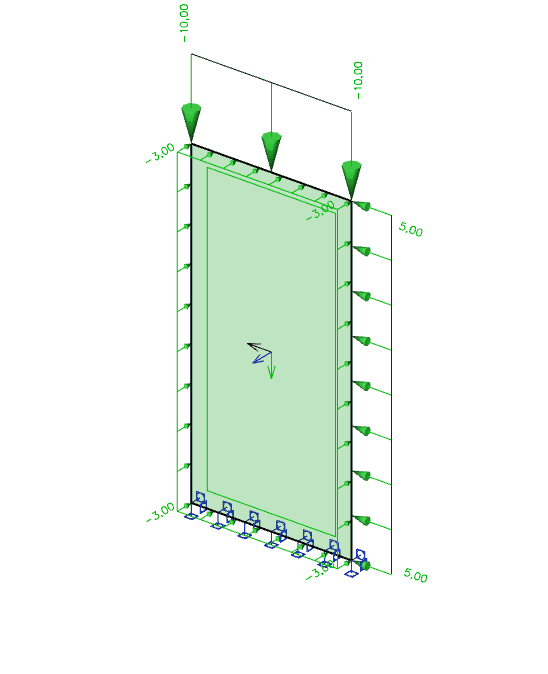# ResultInternalForce2DEdge¶

Internal force on 2D edge

Internal forces on edge of 2D member. The coordinate system of the results is the coordinate system of the member the results belong to.## Specification in the excel¶

Data Type

Example / enum definition

Required

Description

Result on

Enum

On edge

yes

Specify where the result is, only option is ‘on edge’. Prepared for future expansion (on opening edge..)

2D member

String

B1

yes

Reference to the name of 2D member in StructuralSurfaceMember

Edge

Integer

2

yes

The index starting with 1. The order is according to order of “edges” property in StructuralSurfaceMember

Result for

Enum

yes

Specifies from where the result is coming from (from Load Case, Load Combination)

String

LC1

yes, if Result for = Load case

Reference to the name of StructuralLoadCase

String

COM1

yes, if Result for = Combination

Reference to the name of StructuralLoadCombination

Combination key

String

1.35*LC1+1.5*LC2+1.5*LC3+1.5*LC4

no

Allows to define exact combination per result section

Structure of string:
For envelopes and national standard (code) combinations, this column specifies for which exact combination the result is computed

Section at [m]

Double

0.100

yes

X coordinate on the edge(distance from the start node) where the result is located

Index

Integer

1

yes

Index of the section on edge

mx [kNm/m]

Double

3.00

yes

Result value of bending moment mx

my [kNm/m]

Double

3.00

yes

Result value of bending moment my

mxy [kNm/m]

Double

3.00

yes

Result value of torsion moment mxy

vx [kN/m]

Double

3.00

yes

Result value of shear force vx

vy [kN/m]

Double

3.00

yes

Result value of shear force vy

nx [kN/m]

Double

3.00

yes

Result value of membrane force nx

ny [kN/m]

Double

3.00

yes

Result value of membrane force ny

nxy [kN/m]

Double

3.00

yes

Result value of shear force nxy